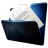# Welcome :-)

I am currently (sept. 2010 - aug. 2011) a post-doctoral fellow at the Laboratoire de Mathématiques Jean Leray, Université de Nantes.

### Research Activities

My current research activity consists of numerical simulations of integral equations and their application to electrocardiology. It involves Boudary Element Method (BEM) and Fast Multipole Method (FMM) from the Finite Element approach.

Last year, I was involved in the making of numerical multi-level model, based on Domain Decomposition technics, for building's phenomena (radiation coupled with advection-diffusion equations).

My PhD deals with Geometric Integration for Partial Differential Equations (namely "Geometric integrators: Application to Fluid Mechanics", see below). The topic could be summarized as follows: assuming that the physical properties of a system are carried by the geometrical structure of the equations (in sense of the differential geometry), specially in their symmetry group, therefore it is natural to expect from numerical methods to preserve the symmetries of the continuous equations. Surprisingly it is in general not the case for most of standard numerical methods! My aim consisted to propose an algorithmic way of construction of such Lie-symmetry-based integrators, their implementation and the applications to Fluid Mechanics.

### Teaching Activities

I have given courses at the University of La Rochelle (from 2005 to 2009) and at the University of Nantes (2010). Most of my courses are related with Numerical Methods, Mathematics and Mechanics.Marx Chhay Professional address: Laboratoire de Mathématiques Jean Leray 2, rue de la Houssinière - BP 92208 F-44322 Nantes Cedex 3, phone: +33 02 51 12 59 32 e-mail: marx _dot_ chhay _at_ univ-nantes _dot_ fr

## Research Activities

• #### My PhD thesis

"Geometric Integrators: Application to Fluid Mechanics"
Dec. 2008, Université de La Rochelle
• #### Publications :

 "Comparison of some Lie-symmetry-based integrators",
M. Chhay, E. Hoarau, A. Hamdouni and P. Sagaut, J. Comput. Phys., 230 (5) pp. 2174-2188, 2011
 "On accuracy of invariant numerical schemes",
M. Chhay and A. Hamdouni, Commun. Pure Appl. Anal. 10(2) pp.761-783, 2011.
 "Lie symmetry preservation by finite difference schemes for the Burgers equation",
M. Chhay and A. Hamdouni, Symmetry. 2, pp. 868-883, 2010.
 "A new construction for invariant numerical schemes using moving frames",
M. Chhay and A. Hamdouni, C. R. Acad. Sci. Meca. 338, pp. 97-101, 2010.
• #### Book Chapter :

 "Symmetry in Turbulence Simulation",
D. Razafindralandy, A. Hamdouni and M. Chhay. in Numerical Simulation Research Progress. Nova Publishers. pp. 161-207, 2009.
• #### Conferences :

• "Invariant numerical schemes (Invited speaker)",
Numerical Methods for Complex Fluid Flows - Wolfgang Pauli Institute. Vienna - Austria, Sept. 21-25, 2009.
• #### Conferences with proceedings:

joint Work with M. Pons. Société Française de Thermique. Perpignan, France, 2011.

• "Un algorithme de construction de schémas invariants par les symétries de Lie",
Colloque SMAI/AUM - Congrès Français de Mécanique. Marseille, France, Aug. 24-28, 2009.

• "Consistency in symmetry for numerical schemes",
International Congress of Theoretical and Applied Mechanics. Adelaide, Australia, Aug. 25-29, 2008.

• "Invariant numerical scheme applied to transfer equation",
European Community on Computational Methods in Applied Sciences - Multiscale computational methods for solid and fluids. Paris-Cachan, France, Nov. 28-30, 2007.

• "Schémas numériques symétrisés pour les équations de transfert",
Congrès Français de Mécanique. Grenoble, France, Aug. 27-31, 2007.

## Teaching Activities

Most of my five years teaching activities concerned the Engineering Formation at the third year of BSc degree (Licence L3) and also at the first year of MSc degree (M1). I was involved in Lectures (L), Recitations (R) and Practical Teaching (PT), including grading activities.

2010-2011 : Vacation (28h - Université de Nantes)
• Mathematics :
• Differential equations and Geometry, R (28h), L2

2008-2009 : 1/2 ATER (96h - Université de La Rochelle)
• Numerical Methods :
• Finite elements in linear elasticity, R (15h), PT (9h), M1
Non-linear structure behaviour and numerical methods, R (15h), M1
Introduction to numerical methods, R (21h), L3
• Mathematics :
• Geometric methods in Mechanics and formal computation, R (10h), M1
Mathematical tools for engineering, R (15h), L3
• Mechanics :
• Vibrations, R (15h), M1
Thin structure dynamics, R (12h), M1
2005-2008 : Monitorat (64h/year - Université de La Rochelle)
• Numerical Methods :
• Introduction to numerical methods, R (21h), L3
• Mathematics :
• Probability ans Statistics, L (18h) , R (24h), L3
Mathematical tools for engineering, R (30h), L3
• Mechanics :
• Real fluid Mechanics, R (24h), L3
Continuous medium Mechanics, R (24h), L3• Thesis folder